# Test Level 1: Electrostatic Potential And Capacitance

## 25 Questions MCQ Test Physics Class 12 | Test Level 1: Electrostatic Potential And Capacitance

Description
This mock test of Test Level 1: Electrostatic Potential And Capacitance for Class 12 helps you for every Class 12 entrance exam. This contains 25 Multiple Choice Questions for Class 12 Test Level 1: Electrostatic Potential And Capacitance (mcq) to study with solutions a complete question bank. The solved questions answers in this Test Level 1: Electrostatic Potential And Capacitance quiz give you a good mix of easy questions and tough questions. Class 12 students definitely take this Test Level 1: Electrostatic Potential And Capacitance exercise for a better result in the exam. You can find other Test Level 1: Electrostatic Potential And Capacitance extra questions, long questions & short questions for Class 12 on EduRev as well by searching above.
QUESTION: 1

### For a charged conductor of arbitrary shape, inside the conductor

Solution:

The electric field on the surface of a hollow conductor is maximum and it drops to zero abruptly inside the conductor. Since
E = - dV\dr

the potential difference between any two points inside the hollow conductor is zero. This means that the potential at all points inside the hollow charged conductor is same and it is equal to the value of the potential at its surface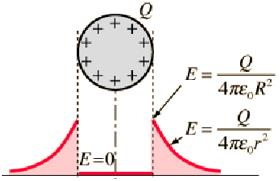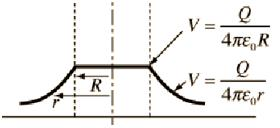QUESTION: 2

### An equipotential surface is a surface

Solution:

When the potential at all points on the surface is the same; such a surface is called an equipotential surface. The potential difference between any two points on an equipotential surface is zero, while the potential may be positive, negative or even zero.

QUESTION: 3

### A variable capacitor and an electroscope are connected in parallel to a battery. The reading of the electroscope would be decreased by

Solution:

An electroscope is a device which measures the potential difference. If it is connected in parallel to the capacitor, the potential across it will be equal to the potential across the capacitor, which is equal to the potential across the battery. On decreasing the battery potential, the potential difference across the electroscope reduces and hence the reading reduces.

QUESTION: 4

A 2μF capacitor C1 is charged to a voltage 100 V and a 4 μF capacitor C2 is charged to a voltage 50 V. The capacitors are then connected in parallel. What is the loss of energy due to parallel connection?

Solution: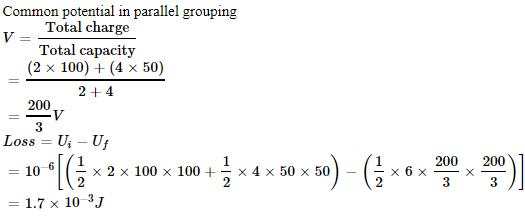QUESTION: 5

If the potential difference between the plates of a capacitor is increased by 0.1%, the energy stored in the capacitor increases by very nearly

Solution:

The intial energy stored in the capacitor ar potential difference V is E = 1/2CV2 when the p.d increased by ΔV, the energy stored is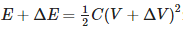solving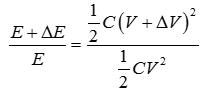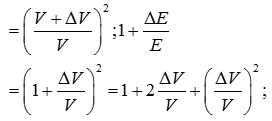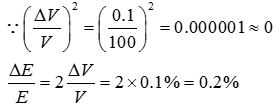QUESTION: 6

If the diameter of earth is 128 x 10km, then its capacitance will be

Solution:

The capacitance of a spherical conductor of radius R is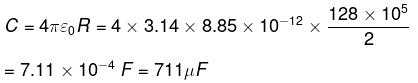QUESTION: 7

If a charge moves in an electrical field

Solution:

When a charged particle moves in an electric field, if its electric potential energy decreases, its kinetic energy will increase. In other words, the total energy is conserved.

QUESTION: 8

If the charge on a capacitor is increased by 2 coulomb, the energy stored in it increases by 21%. The original charge on the capacitor (in coulomb) is

Solution:

The initial energy of the capacitor of capacitance C and charge Q1 is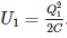.When the charge increases to Q2 Qi the energy of the capacitor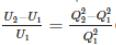Given percentage increase of energy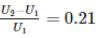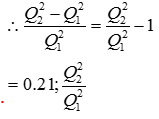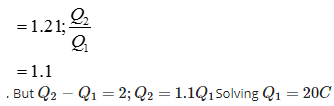QUESTION: 9

In series combination of capacitors, potential drops across the individual capacitors are equal to

Solution:

When capacitors are connected in series, they have equal charge but the potential difference across them is given by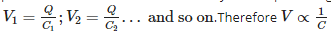QUESTION: 10

A capacitor of capacitance C is fully charged by a 200 V supply. It is then discharged through a small coil of resistance wire embedded in a thermally insulated block of specific heat 2.5×102Jkg−1K−1 and of mass 0.1 kg. If the temperature of the block rises by 0.4 K, what is the value of C?

Solution:

Energy stored in the capacitor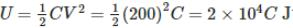This is released as heat when the capacitor discharges through the metal block. The quantity of heat = mass xs p.heatx rise in temperature.
Q = m x s x Δθ = 0.1 x 2.5 x 102 x 0.4 = 10 J
U = Q; 2 x 104 C = 10; C = 5 x 10-4 F = 500 μF

QUESTION: 11

64 water drops having equal charges combine to form one bigger drop. The capacitance of the bigger drop, as compared to that of smaller drop will be

Solution:

Capacitance of the small drop of radius r C8 = 4πε0r  and that of the big drop of radius R is CB = 4πε0R .  The volume of 64 small drops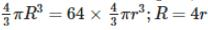The ratio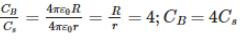QUESTION: 12

An electrolytic capacitor is marked 8 μF, 220 V. It can be used in a circuit where the p.d. across the capacitor may be

Solution:

The break down potential of the capacitor is 220 V. In order to prevent damage to a capacitor, it should be always used in a circuit where the p.d is less than its break down potential. The p.d difference can only be 200 V.

QUESTION: 13

The capacitance of a parallel plate capacitor is 5 μF. When a glass slab of thickness equal to the separation between the plates is introduced between the plates, the potential difference reduces to 1/8 of the original value. The dielectric constant of glass is

Solution:

When a dielectric of constant K is introduced between the plates of a capacitor, the potential V reduces to V/K. Therefore K=8

QUESTION: 14

For a parallel plate capacitor ______________ possible potential difference between the capacitor plates

Solution:

When a dielectric is introduced between two charged plates of a capacitor having a charge Q and maintained at a potential difference of V, a reverse electric field is set up inside the dielectric due to dielectric polarization.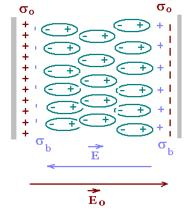This reduces the electric field in between the plates. The potential is also reduced. Maximum potential is dependent on the charge on the plates. As the charge remains constant, the presence of the dielectric decreases the maximum potential between the plates.

QUESTION: 15

Which of the following is true about equipotential lines

Solution:

The potential at all points on an equipotential surface is constant and work done in moving a charge on an equipotential surface is zero. Electric field lines show the direction of the electric field at the point. If the electric field lines were tangential, parallel or opposite to the equipotential surface, a tangential field will exist on the surface and work done in moving a charge on the surface is not zero. Therefore electric field lines are always perpendicular to the equipotential surface.

QUESTION: 16

In electrolytic capacitors positive terminal is

Solution:

Aluminium electrolytic capacitors have the Aluminium foil anode (positive terminal) which is attached and covered with a layer of Aluminium Oxide which acts as a dielectric. The whole assembly is covered using a paper separator soaked in electrolyte such as, Borax or Glycol and covered by Aluminium foil which acts as cathode ( negative electrode)

QUESTION: 17

If a positive charge moves opposite to the direction of the electric field

Solution:

A positive charge experiences a force in the direction of the field. If it moves opposite to the direction of the field, negative work is done by the electric field on the charge. Potential energy of the charge increases since an external source is required to do work on the charge against the direction of the field, and the work done is stored in the charge as its potential energy.

QUESTION: 18

Submarine cables act as

Solution:

A submarine cable consists of an inner conductor which carries power. This conductor is covered by an insulator, which acts as a dielectric. The dielectric material is covered by a metal coating called shield, which is connected to ground.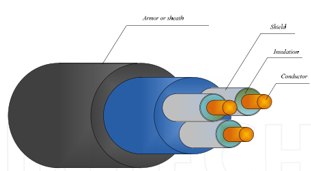The cable acts as a cylindrical capacitor, with the conductor acting as the inner cylinder, and the metal shield as the outer cylinder which is connected to earth.

QUESTION: 19

When 2 charged conductors are connected by a wire,

Solution:

Let the two conductors have capacitances C1 and C2 and let their potentials be V1 and V2 . Total initial energy of the conductors Ui=1/2C1V12+1/2C2V22. When two charged conductors are connected by a wire, the flow of charge stops when the potentials are equalized. Let V be the common potential and Q1 and Q2 be the charges on the conductors after the common potential is attained.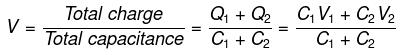The final energy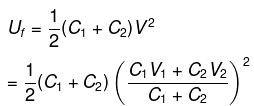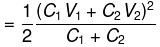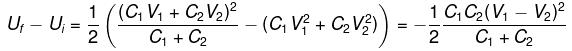QUESTION: 20

Electrostatic shielding is based

Solution:

Electric field inside a cavity is always zero. The potential inside a cavity of a conductor is constant. The potential difference between any two points inside a charged conductor is zero. This phenomenon is called electrostatic shielding.

QUESTION: 21

Two metallic spheres of radii 1 cm and 3 cm are given charges of (-1 x 10-2 C) and 5 x 10-2 C , respectively. If these are connected by a conducting wire, the final charge on the bigger sphere is

Solution:

Charge flows from high potential to low potential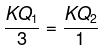Q1 = 3Q2
also,
Q1 + Q2 = 4 x 10-2
4Q2 = 4 x 10-2
Q2 = 10-2
Q1 = 3 x 10-2 C

QUESTION: 22

Consider the situation shown in the figure. The capacitor A has a charge q on it whereas B is uncharged. The charge appearing on the capacitor B a long time after the switch is closed is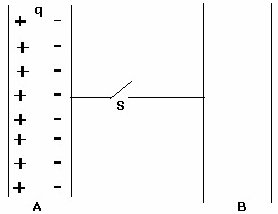Solution:

The charge –q is bound to the charge +q. Therefore no charge will appear on the capacitor B. The capacitor B will acquire a charge only if a battery ( driving force) is put in the circuit.

QUESTION: 23

Three capacitors, each of capacitance C = 3 mF, are connected as shown in the figure. The equivalent capacitance between points P and S is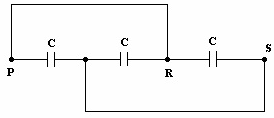Solution: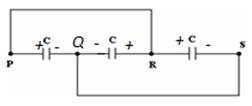If P is at positive potential, then Q is at negative potential and R is at positive potential. The system therefore reduces to 3 capacitors in parallel. C= 9μF

QUESTION: 24

When two capacitors are put in series, the equivalent capacitance is

Solution:

When two capacitors C1 and C2 are connected in series, the reciprocal of the equivalent capacitance in series is equal to the sum of the reciprocals of the two individual capacitances.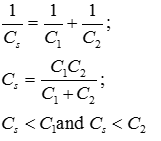It has value lesser than the least value of capacitance.

QUESTION: 25

When a capacitor is connected to a battery

Solution:

When an uncharged capacitor is connected to a battery, charges flow from the poles of the battery to the plates of the capacitor and this process continues till the potential across the capacitor attains the potential difference of the battery. The current flows in the circuit till the time the capacitor is charged and then it ceases.